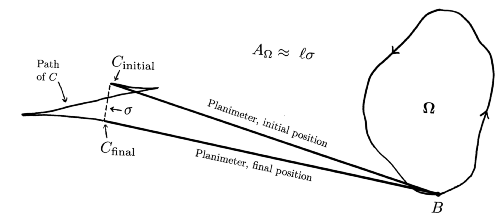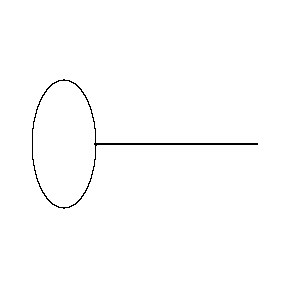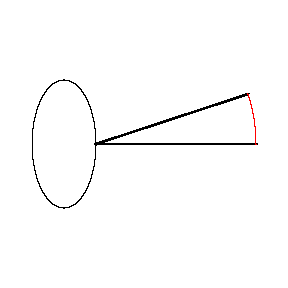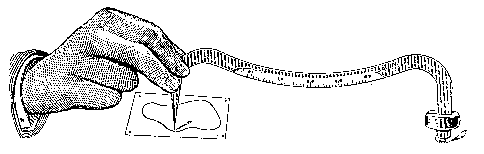## Using a Prytz Planimeter to Measure Area

Area is measured, but only approximately.  When the tracer point T moves around a closed curve, the chisel edge C follows a zig-zag path.  When the tracer point returns to the base point B, the chisel edge C has undergone a net rotational displacement s.  Multiply s by the length l of the planimeter and you get the area of the region!If this sounds too good to be true, you're right, but the error has a very nice geometric interpretation.  As the tracer point goes counterclockwise around the boundary of the region W being measured starting and stopping at B, the chisel edge C traces parts of two nearly triangular regions.  The the circular arc of length s forms the remaining sides of these triangles.  Note that C traces the upper triangle in the clockwise (CW) direction and the lower one in the counterclockwise (CCW) direction.  It turns out that the product ls equals the area A of W plus the area of the CW triangle minus the area of the CCW triangle.  In symbols (and solved for AW),

AW  =  ls + ACCW  - ACW .                            Why this is true.

It follows that the error made by using ls as an approximation for the area of W is the difference between the areas of these triangular regions.  By carefully choosing the starting position of the planimeter, you can measure areas fairly accurately with this simple device!  It also turns out that the error is inversely proportional to the length of the planimeter, so measuring a small region with a large planimeter is more accurate.

Here is an ellipse with major diameter 4 and minor diameter 2 being measured by a planimeter of length 5.  The picture on the right shows the initial and final positions of the planimeter, and the circular arc between them (in red).The length of the circular arc is about 1.59.  Multiplying by the length of the planimeter yields 7.94 as an approximation of the area of the ellipse.  The real area is, of course, 2pi, or about 6.28, so the approximation is a whopping 26% too big!  Measuring the ellipse with a longer planimeter gives a better approximation.  For example, a planimeter of length 10 gives an approximation of 7.00 for the area, which is 11% too big.  One of length 20 gives an approximation of 6.62 for the area, only 5.3% too big.

Changing the initial direction of the planimeter makes a difference in the result.  If the planimeter of length 5 starts pointing down instead of to the right (still starting at the same point on the ellipse), the approximation for the area is 6.77, which is only 7.8% too large.  If it starts pointing to the left, the approximation for the area is 5.23, which is 17% too small.

Goodman's variation.  When using the planimeter, you usually just measure the straight-line distance between the initial and final positions of the chisel edge C, instead of the actual arc-length.  In 1896 an engineer named Goodman designed a variation of Prytz's planimeter by incorporating a curved scale into the bar.  The radius of the curve equals the length of the planimeter, and so the arc-length between the inital and final positions of the chisel can be measured directly from this scale.  This, however, does not correct the inherent inaccuracy of the instrument.
Variation by GoodmanPhoto of an actual Goodman planimeter

 Main Prytz planimeter page Pictures of Prytz Planimeters Animations of Prytz planimeters Last update 14 January 2004 Robert Foote